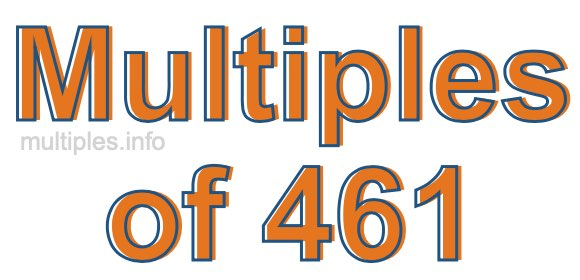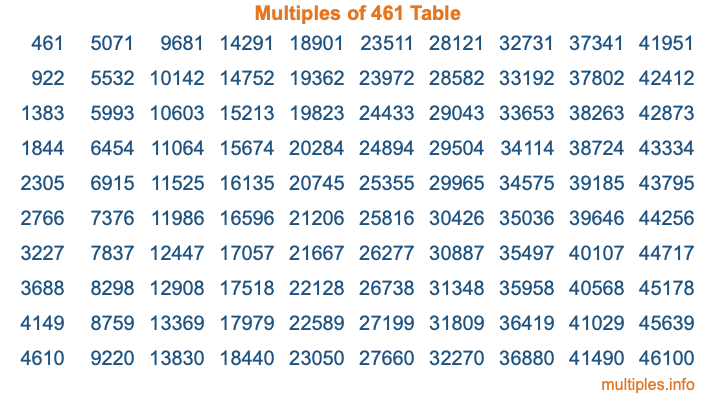Multiples of 461Welcome to the Multiples of 461 page. Here we will first teach you everything you will ever need to know about the multiples of 461, and then give you a study guide summary of everything we taught you to make sure you remember it all. Use this page to look up facts and learn information about the multiples of 461. This page will make you a multiples of four hundred sixty-one expert!

Definition of Multiples of 461
Multiples of 461 are all the numbers that when divided by 461 equal an integer. Each of the multiples of 461 are called a multiple. A multiple of 461 is created by multiplying 461 by an integer.

Therefore, to create a list of multiples of 461, you start with 1 multiplied by 461, then 2 multiplied by 461, then 3 multiplied by 461, and so on for as long as you want. Thus, the list of the first five multiples of 461 is 461, 922, 1383, 1844, and 2305. To see a larger list of multiples of 461, see the printable image of Multiples of 461 further down on this page. We also have a category where you can choose any nth multiple of 461.

Multiples of 461 Checker
The Multiples of 461 Checker below checks to see if any number of your choice is a multiple of 461. In other words, it checks to see if there is any number (integer) that when multiplied by 461 will equal your number. To do that, we divide your number by 461. If the the quotient is an integer, then your number is a multiple of 461.

Is  a multiple of 461?

Least Common Multiple of 461 and ...
A Least Common Multiple (LCM) is the lowest multiple that two or more numbers have in common. This is also called the smallest common multiple or lowest common multiple and is useful to know when you are adding our subtracting fractions. Enter one or more numbers below (461 is already entered) to find the LCM.

Check out our LCM Calculator if you need more details about the Least Common Multiple or if you need the LCM for different numbers for adding and subtraction fractions.

nth Multiple of 461
As we stated above, 461 is the first multiple of 461, 922 is the second multiple of 461, 1383 is the third multiple of 461, and so on. Enter a number below to find the nth multiple of 461.

th multiple of 461

Multiples of 461 vs Factors of 461
461 is a multiple of 461 and a factor of 461, but that is where the similarities end. All postive multiples of 461 are 461 or greater than 461. All positive factors of 461 are 461 or less than 461.

Below is the beginning list of multiples of 461 and the factors of 461 so you can compare:

Multiples of 461: 461, 922, 1383, 1844, 2305, etc.

Factors of 461: 1, 461

As you can see, the multiples of 461 are all the numbers that you can divide by 461 to get a whole number. The factors of 461, on the other hand, are all the whole numbers that you can multiply by another whole number to get 461.

It's also interesting to note that if a number (x) is a factor of 461, then 461 will also be a multiple of that number (x).

Multiples of 461 vs Divisors of 461
The divisors of 461 are all the integers that 461 can be divided by evenly. Below is a list of the divisors of 461.

Divisors of 461: 1, 461

The interesting thing to note here is that if you take any multiple of 461 and divide it by a divisor of 461, you will see that the quotient is an integer.

Multiples of 461 Table
Below is an image of the first 100 multiples of 461 in a table. The table is in chronological order, column by column. The first column has the first ten multiples of 461, the second column has the next ten multiples of 461, and so on.The Multiples of 461 Table is also referred to as the 461 Times Table or Times Table of 461. You are welcome to print out our table for your studies.

Negative Multiples of 461
Although not often discussed or needed in math, it is worth mentioning that you can make a list of negative multiples of 461 by multiplying 461 by -1, then by -2, then by -3, and so on, to get the following list of negative multiples of 461:

-461, -922, -1383, -1844, -2305, etc.

Multiples of 461 Summary
Below is a summary of important Multiples of 461 facts that we have discussed on this page. To retain the knowledge on this page, we recommend that you read through the summary and explain to yourself or a study partner why they hold true.

There are an infinite number of multiples of 461.

A multiple of 461 divided by 461 will equal a whole number.

461 divided by a factor of 461 equals a divisor of 461.

The nth multiple of 461 is n times 461.

The largest factor of 461 is equal to the first positive multiple of 461.

461 is a multiple of every factor of 461.

461 is a multiple of 461.

A multiple of 461 divided by a divisor of 461 equals an integer.

461 divided by a divisor of 461 equals a factor of 461.

Any integer times 461 will equal a multiple of 461.

Multiples of a Number
Here you can get the multiples of another number, all with the same attention to detail as we did for multiples of 461 on this page.

Multiples of
Multiples of 462
Did you find our page about multiples of four hundred sixty-one educational? Do you want more knowledge? Check out the multiples of the next number on our list!